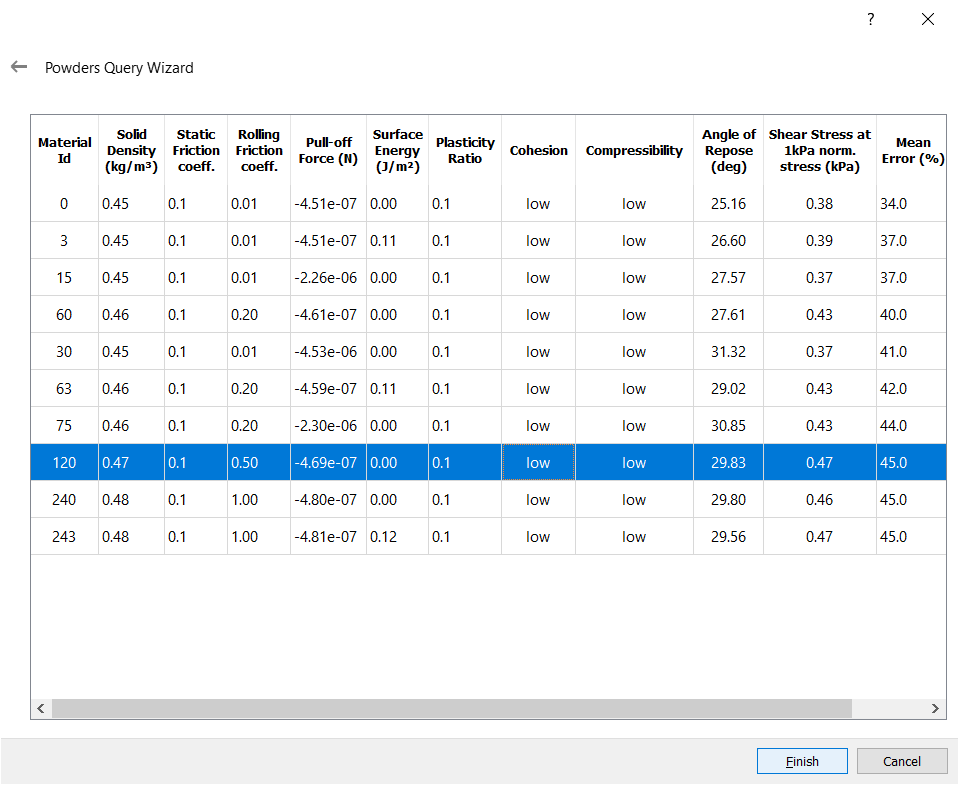# EDEM Powders Database

The EDEM powders database is a collection of EDEM material models for powders that includes results for the static angle of repose and the steady-state shear stress responses described in Figure 1. The physical measurement of the static angle of repose can be conducted according to ASTM 6393 or similar and the measurement of the steady state shear stress corresponds to the pre-shear stage of a shear cell test performed according to ASTM 6128 or ASTM D6773. Steady-state shear stress results are available for 1kPa and 5kPa normal stresses.

a)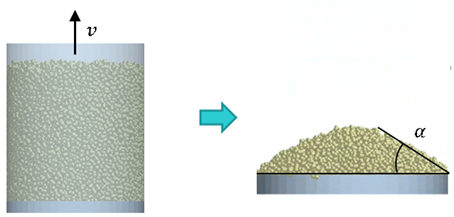b)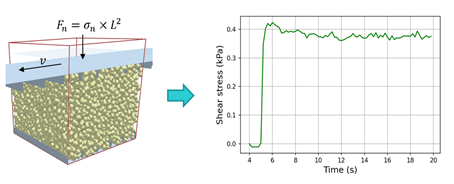Figure 1 a) Angle of repose test via the lifting cylinder method and b) direct shear test under constant normal stress

A meso-scopic modelling approach is adopted for the EDEM powder models in the interest of computational efficiency.

The two-sphere particle shape shown in Figure 3 and the normal relative particle size distribution described in Table 1 are adopted to capture shear dilation effects and avoid non-physical packing states (Härtl, J., & Ooi, J. Y., 2011). The standard particle diameter in the database D_max is 1.5 mm but different values can be used in certain cases and these are discussed in section 1.2.

The interaction forces at the meso-contact scale are described with the Edinburgh Elastic-Plastic-Adhesive (EEPA) and Type C rolling friction models. The model parameter space is listed in Table 1.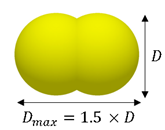Figure 2 Numerical particle shape

 Particle size distribution Type Dmin/D50 Dmax/D50 σ/D50 Normal 0.85 1.15 0.15 Fixed parameter space Particle Poisson’s ratio v 0.25 Particle shear modulus G  (Pa) 1e+7 Particle solid density ρs (kg/m3) 1000 Particle coefficient of restitution e 0.1 Particle – geometry coefficient of static friction μg 0.5 Particle – geometry coefficient of rolling friction μrg 0.1 EEPA tangential stiffness multiplier ktan 0.667 EEPA loading exponent n 1 EEPA tensile exponent kadh 3 Variable parameter space Particle – particle coefficient of static friction μs Particle – particle coefficient of rolling friction μr EEPA constant pull-off force f0 EEPA surface energy γ (J/m2) EEPA contact plasticity ratio λ
Table 1 Model parameter space

The database contains 1500 material models. The probability density distributions for the responses are shown in Figure 3.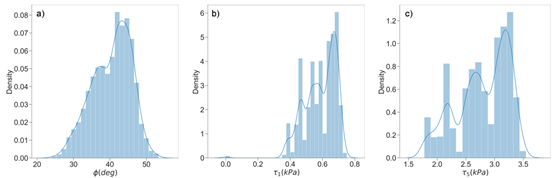Figure 3 Probability density distribution for a) the static angle of repose, b) the steady state shear stress at 1 kPa normal stress and c) the steady state shear stress at 5 kPa normal stress.

The database can be searched by multiple responses simultaneously and the results are sorted by the total relative error between the requested response values and the results in the database. The required inputs for the search are:

• The bulk cohesion and compressibility, which are qualitatively categorized using the bond number and the EEPA contact plasticity ratio respectively as described in Figure 4.
• The bulk density, which his captured via particle solid density and Bond number scaling of the baseline values in the dataset and has variable ranges for the different bulk cohesion levels.
• The mean particle diameter, which is available as an input if the search includes only steady state shear responses. The scaling rules proposed by Thakur et. al are applied to compute the relevant parameter values in this case.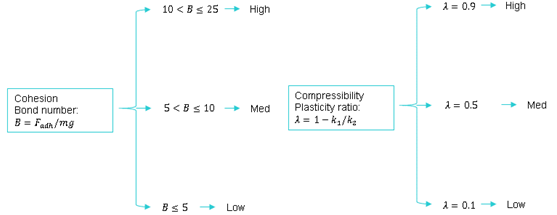Figure 4 Bulk cohesion and compressibility categorization in the EDEM powders database.

## EDEM Powders Database

The Powders Database can be access from Bulk Materials>Select Materials Wizard>Open Powders Wizard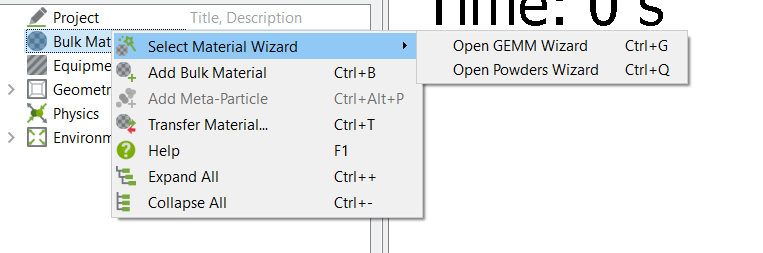Progress through the database to the input screen where in the input parameters for the soils database should be entered.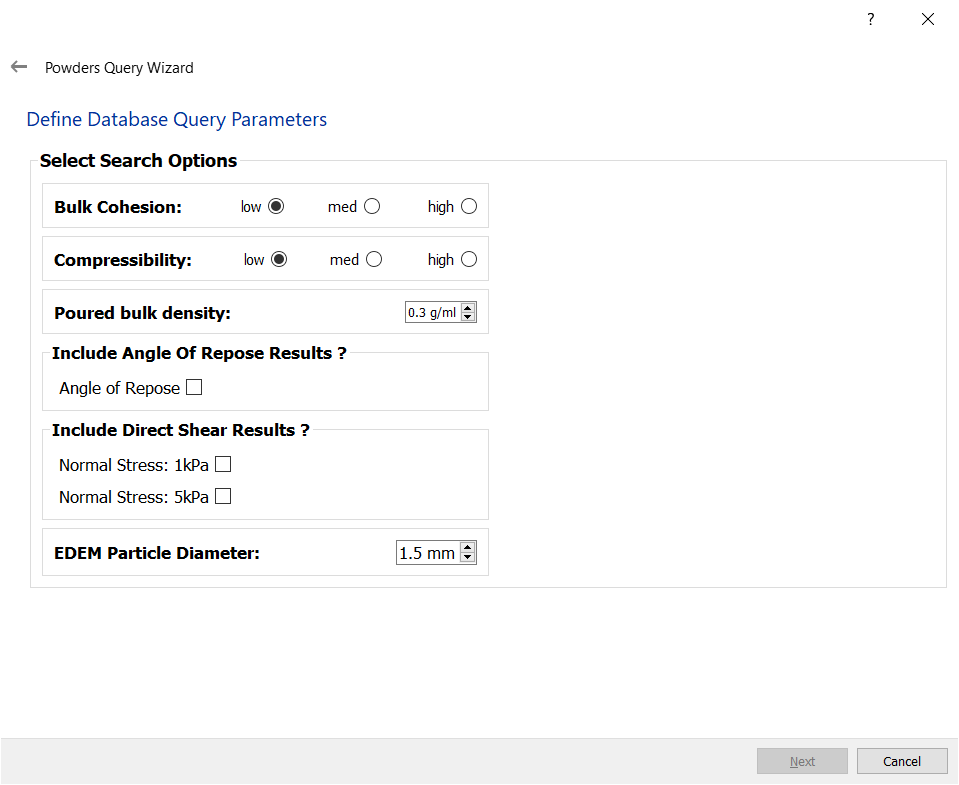Finally a range of materials appears, selecting an appropriate material will populate the material and model parameters in the EDEM GUI.